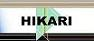| Home | Journals | Books | Paper submission | About us and our mission | News | Contact us |Site Search:

## Online Edition, Vol. 3, 2009, no. 9-12

G. M. Gharib
Conservation laws for the Calogero-Degasperis family of equations which describe pseudo-spherical surfaces
Int. Journal of Math. Analysis, Vol. 3, 2009, no. 9-12, 399-411.

Haiqing Wang
Periodic solutions for higher order delay functional differential equation with complex deviating argument
Int. Journal of Math. Analysis, Vol. 3, 2009, no. 9-12, 413-418.

K. El-Hussein
Eigendistributions for the invariant differential operators on the affine group
Int. Journal of Math. Analysis, Vol. 3, 2009, no. 9-12, 419-429.

Nina Shang, Youmin Lu, Fuyi Xu
Oscillating criteria on second order non-linear differential equations with distributed deviating arguments and delay terms
Int. Journal of Math. Analysis, Vol. 3, 2009, no. 9-12, 431-441.

M. H. Dida, F. Hathout, M. Djaa
On the geometry of the second order tangent bundle with the diagonal lift metric
Int. Journal of Math. Analysis, Vol. 3, 2009, no. 9-12, 443-456.

Myung Jae Kim
Conditional Fourier-Feynman transform and convolution product on a function space
Int. Journal of Math. Analysis, Vol. 3, 2009, no. 9-12, 457-471.

M. Bekkar, H. Zoubir
Minimal surfaces of the 3-dimensional Lorentz-Heisenberg space
Int. Journal of Math. Analysis, Vol. 3, 2009, no. 9-12, 473-482.

A. Sofo
Double integral representation of sums
Int. Journal of Math. Analysis, Vol. 3, 2009, no. 9-12, 483-489.

Asymptotic formulas for the number of negative eigenvalues of a differential operator with operator coefficent
Int. Journal of Math. Analysis, Vol. 3, 2009, no. 9-12, 491-504.

M. Terbeche, B. O. Oluyede
On some differential equations
Int. Journal of Math. Analysis, Vol. 3, 2009, no. 9-12, 505-526.

R. Kumar, R. K. Nagaich
Indefinite RK-manifolds of constant holomorphic sectional curvature
Int. Journal of Math. Analysis, Vol. 3, 2009, no. 9-12, 527-534.

Nguyen Buong, Lam Thuy Duong
Regularization auxiliary problem algorithm for common fixed points of a countably infinite family of non-self strictly pseudocontractive mappings
Int. Journal of Math. Analysis, Vol. 3, 2009, no. 9-12, 535-547.

Nguyen Buong, Truong Minh Tuyen
Regularization inertial proximal point algorithm for convex feasibility problems in Banach spaces
Int. Journal of Math. Analysis, Vol. 3, 2009, no. 9-12, 549-561.

H. Chandra, A. Bhatt
Fixed point theorems for occasionally weakly compatible maps in probabilistic semi-metric space
Int. Journal of Math. Analysis, Vol. 3, 2009, no. 9-12, 563-570.

E. D. Nayeri, D. Behmardi
Investigation of some topological points
Int. Journal of Math. Analysis, Vol. 3, 2009, no. 9-12, 571-574.

Y. Abilov, M. Acikgoz
Banach spaces and extreme points
Int. Journal of Math. Analysis, Vol. 3, 2009, no. 9-12, 575-579.

Jingli Xie, Guoping Chen, Xiaofei He
Integral boundary value problems for first order impulsive differential inclusions
Int. Journal of Math. Analysis, Vol. 3, 2009, no. 9-12, 581-590.

Than Sint Khin, Ning Su
Propagation property for nonlinear parabolic equations of p-Laplacian-type
Int. Journal of Math. Analysis, Vol. 3, 2009, no. 9-12, 591-602.

I. D. Arandelovic, M. Rajovic, V. Kilibarda
On the convergence of Ishikawa iterates defined by nonlinear quasi-contractions
Int. Journal of Math. Analysis, Vol. 3, 2009, no. 9-12, 603-610.